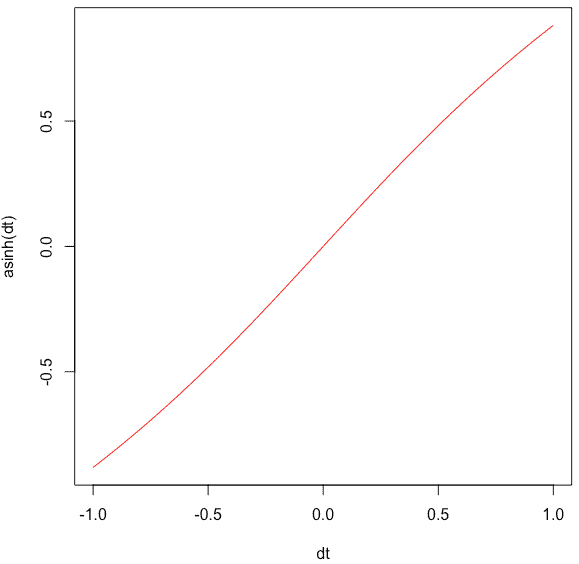# asinh in R: How to Use asinh() Function in R

In mathematics, the inverse hyperbolic functions are the inverse functions of the hyperbolic functions. The acosh(x) returns the inverse hyperbolic cosine of the elements of x when x is a REAL scalar, vector, matrix, or array. The result has the same shape as x. The inverse hyperbolic cosine function is defined by x == cosh(y).

## asinh in R

The asinh() is a built-in R function that calculates the inverse hyperbolic sine of a number. The inverse hyperbolic sine is the value whose hyperbolic sine is the number.

To calculate the hyperbolic arcsine in R, use the asinh() function.

Syntax

``asinh(x)``

### Parameters

x: It is a numeric value, array, or vector.

### Example

Let’s calculate the asinh value of 1 and 0.

``````asinh(0)
asinh(1)``````

#### Output

`````` 0
 0.8813736``````

## Calculate asinh() of complex number

Define a complex value and pass that value to the asinh() function.

``````dt <- 8 + 9i
asinh(dt)``````

#### Output

`` 3.181316+0.842441i``

## Plotting the asinh() function to a graph

We can use the seq() function to create a series of values and pass that to the plot() function, which will create a line chart.

``````dt <- seq(-1, 1, by = 0.01)
plot(dt, asinh(dt), type = "l", col = "red")``````

#### OutputThe function returns the NaN value that is why it can’t draw a graph based on that value.

## Applying asinh() function to a Vector

To create a Vector in R, use the c() function. Then pass that vector to the asinh() function.

``````rv <- c(-1, 0.5, 0, 0.5, 1)
asinh(rv)``````

#### Output

`` -0.8813736 0.4812118 0.0000000 0.4812118 0.8813736``

## Passing a pi to the asinh() function

The pi is an inbuilt constant in R programming, and its value is 3.141593.

Let’s find the pi constant’s asinh() value.

``asinh(pi)``

#### Output

`` 1.862296``

Let’s see another example of pi.

``asinh(pi / 4)``

#### Output

`` 0.7212255``

That is it for this tutorial.